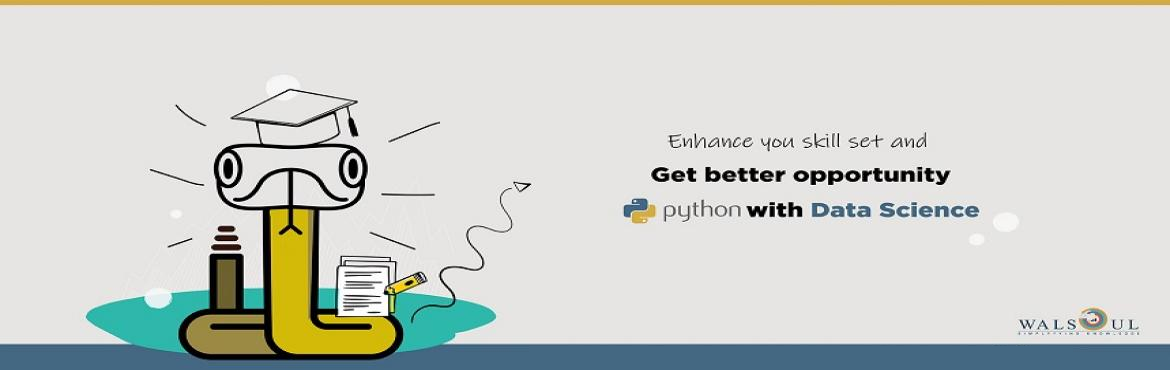# Data Science with Python

• ## Online & Offline

Sale Date Ended

INR 25000
Sold Out

#### Invite friends

Page Views : 35

Python Basic :

• Introduction to Python
• Running Python from eclipse
• IDLE
• Data type in Python
• Integer
• Long
• Float
• Complex
• None
• Typecasting data
• Operators in python

Collections  in  python:

• List

◦     Introduction to List

◦     List operations

◦     List Comprehensions

• Dictionary

◦     Introduction to Dictionary

◦     Dictionaries operations

• Set

◦     Introduction to Set

◦     Set Operations

• Tuples

◦     Introduction to Tuples

◦     Tuples Operations

Conditionals and Looping

• Introduction to conditionals
• if
• if and else
• if elif and else
• Introduction to looping
• for loop
• while loop
• break
• continue
• pass

Strings:

• Introduction to Strings
• String Operations

Functions modules and Package:

• Inbuilt Functions
• User Defined Functions and its definition
• return statement
• Local and global variables
• Default argument
• Variable length arguments
• Anonymous functions
• Modules in Python
• Packages in python
• Writing packages
• importing packages

Exception handling in python :

• Introduction to exception
• Raising Exception
• try and except
• Different type of exception
• Multiple except
•  Role of finally
• try, except and finally together
• assert

IO in python

• Reading from and writing to console.
• Writing to a file
• Reading and Writing csv (Comma Separated Files)
• Reading and Writing JSON files.
• Reading and writing excel files.

Some useful packages in Python

• os

◦     Joining path

◦     Creating new directory

◦     Absolute and relative path

◦     File size

◦     Getting content of a folder

• shutil

◦     Copying files and directories

◦     Deleting files and directories

◦     Renaming files and directories

Class and Objects :

• Class in Python
• Constructors
• Objects
• Inheritance
• Namespace

Debugging in Python

NumPy :

• Introduction to NumPy.
• NumPy data type
• NumPy array and its operations
• NumPy ndarray
• Indexing slicing and Stacking of array and ndarray
• Manipulating array shapes
• Splitting arrays
• Matrices and its operation in NumPy
• Linear systems and NumPy
• File IO in NumPy

Matplotlib :

• Introduction to Matplotlib.
• Line plot multiple line plot
• Scatter plot
• Bar plot
• Histograms
• Box plot
• Error bars
• Contour plot
• Piplot
• Different aspects of coloring
• Violin plots
• Text plot
• Word Cloud

NLTK :

• Introduction to natural language processing.
• Introduction to NLTK
• Installing NLTK
• Text analysis basics

◦     Tokenization

◦     Stemming

◦     Stop words

◦     Part of speech tagging

◦     Lemmatization

• NLTK Corpora
• Clustering

Pandas :

• Introduction to Pandas.
• Pandas data structure
• Operations on Data structures
• IO in Pandas
• Data summarization and aggregation

Scikit-Learn

Introduction :

• Introduction to Data Mining and Machine learning
• Supervised and unsupervised learning.
• Some use cases on machine learning.
• Introduction to Scikit-Learn
• Installing Scikit-Learn
• What is data?
• Steps in data analysis.
• Types of data.
• Data preprocessing and its importance.

Exploratory analysis of data :

• Importance of data visualization
• Initial questions in model building.
• Model selection

Linear regression

• Introduction to simple linear regression.
• Assumption to simple linear regression.
• Parameter estimation
• Simple linear regression with Scikit-Learn
• Multiple linear regression with Scikit-Learn
• Model verification and linear regression assumption testing.
• Data transformation and polynomial regression
• Introduction to Ridge regression
• Ridge regression and Scikit Learn
• Introduction to Lasso Regression
• Lasso Regression and Scikit Learn

Classification

• Introduction to classification
• Classification use cases
• K Nearest neighbor

◦     Introduction to K Nearest neighbor

◦     K Nearest neighbor in Scikit Learn

◦     Strength and weakness of K Nearest neighbor

• Logistic Regression

◦     Introduction to logistic regression

◦     Use cases of logistic regression

◦     Mathematical description of logistic regression

◦     Logistic regression with Scikit Learn

• Naive Bayes Classifier

◦     Introduction to Bayes theorem

◦     Bayes theorem in classification

◦     Bayes classifier with Scikit Learn

• Decision Tree

◦     Introduction to decision tree.

◦     Use cases of decision tree

◦     Partition algorithms for decision tree

▪     ID3

▪     Gini Index

▪     Cart

◦     Tree pruning

◦     Scikit Learn and decision tree

• Ensemble methods
• Random forest with Scikit Learn
• Neural Networks

◦     Introduction to brain

◦     Introduction to neural network

◦     Perceptron

◦     Back propagation algorithm

◦     MLP and Scikit Learn

• Classifier performance evaluation

◦     Confusion matrix

◦     Cohen kappa

◦     Precision, recall and F-measures

Clustering

• Introduction to clustering
• Use cases of clustering
• K-means clustering
• Hierarchical clustering

• DBSCAN
• Clustering performance evaluation

◦     Mutual Information based scores

◦     Homogeneity, completeness and V-measure

◦     Fowlkes-Mallows scores

◦     Silhouette Coefficient

NLTK :

• Introduction to natural language processing.
• Introduction to NLTK
• Installing NLTK
• Text analysis basics

◦     Tokenization

◦     Stemming

◦     Stop words

◦     Part of speech tagging

◦     Lemmatization

• NLTK Corpora
• Clustering

Projects  :

There will be three projects, which will move end to end .

Project1 : Given sells data, participants has to implement data science day to day algorithm like filtering, aggregation, date and time manipulation and applying charts to understand patterns in sells of different stores.

Project 2 : Given Movie lens data, participant has to implement data joining, aggregation and charting algorithms to find meaningful patterns and informations.

Project 3 : Given Kaggle titanic data, participants have to implement  data preprocessing and data cleaning algorithms. After that, participants are required to do hypothesis testing on the data to validate their hypothesis.